# python————day01 test

1. 在Linux电脑上安装python，ipython，pycharm专业版本软件;

1)在rh镜像中有集成的python安装包 可直接安装（2.x版本），如需更高版本 可在官网自助下载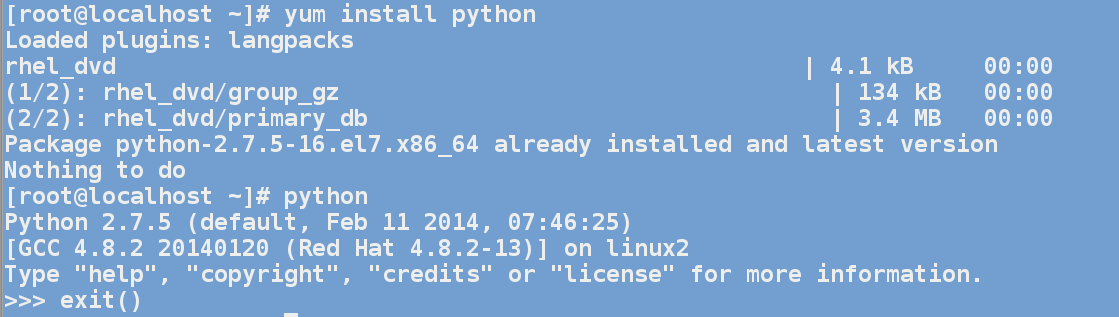2)下载所需ipython按转包 按如下安装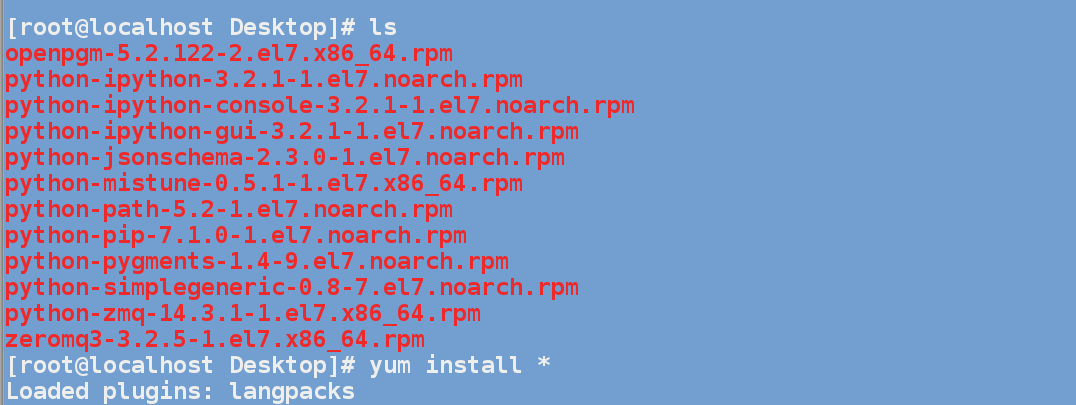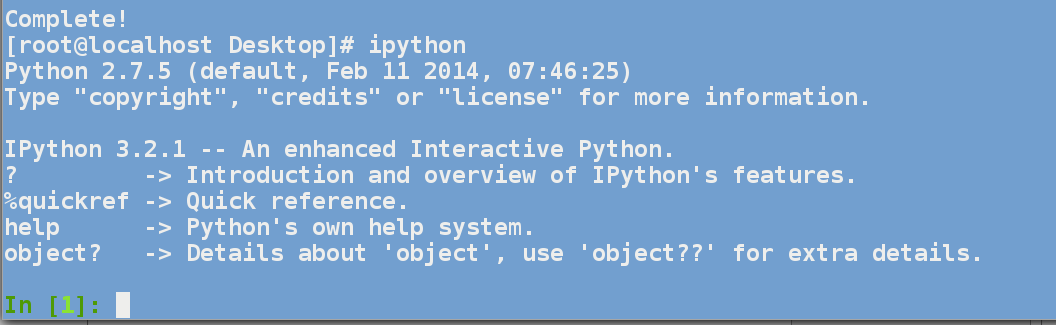3）

2. 在Windows电脑上安装python3版本,并配置环境变量，确保Dos环境下运行脚本;

3. Linux下有多少种运行python的不同方法，并分析各自优缺点。并说说你最喜欢哪一种?

4. 编写脚本，使用print语句编写脚本在屏幕上显示你名字、年龄、最喜欢的颜色

#!/usr/bin/env python
#coding:utf-8

print '''
张璐
永远18谢谢
可能年纪大了吧 喜欢红色黄色粉色
喜欢做甜点吃零食
近期的愿望希望不挂科顺利毕业
梦想能到90斤：）

'''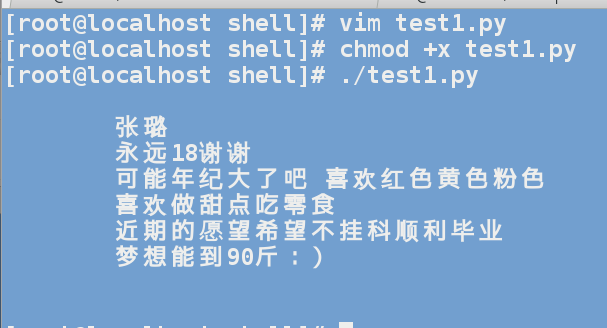5. 阅读下面的 Python 脚本:
#!/usr/bin/env python
1 + 2 * 4

a) 你认为这段脚本是用来做什么的?

计算1+（2*4）的结果

b) 你认为这段脚本会输出什么?

输出计算结果

c) 输入以上代 码,并保存为脚本,然后运行它。它所做的与你的预期一样吗?为什>么一样/不一样?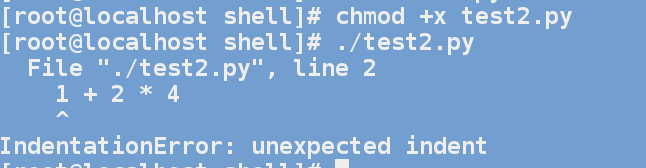不一样

没有定义输出数据类型

d) 这段代码单独执行和在交互解释器中执行有何不同? 试一下,然后写出结果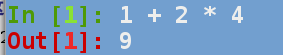e) 如何改进这个脚本, 以便它能和你想像的一 样工作?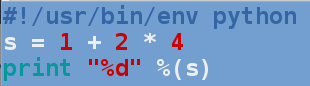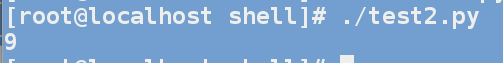6. 变量赋值:
1). 赋值语句 x, y, z = 1, 2, 3 会在 x、y、z 中分别赋什么值?

xyz分别赋值整型数1，2，3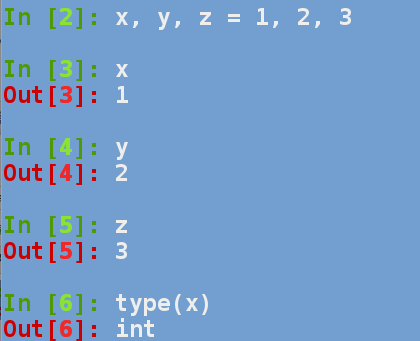2). 执行 z, x, y = y, z, x 后,x、y、z 中分别含有什么值?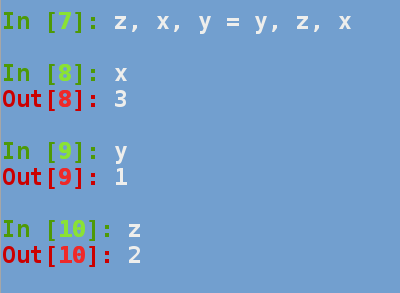7. 标识符。下面哪些是 Python 合法的标识符?如果不是,请说明理由!

int32           40XL            $aving$                 printf          print
_print          this            self                    __name__        0x40L
bool            true            big-westos              2hot2ls         type
if                      do                      counter-1               access          _

int32                                  printf          print
_print          this            self                    __name__
bool            true            big-westos                      type
if                      do                      counter-1               access          _

8. 带循环和条件判断的给定一个数值num, 用户输入使用raw_input()函数来提示用户输入一个1和100之间的数,如果用户输入的数等于num, 显示成功并退出。否则显示一个错误信息，然后再次提示用户输入数值,直到满足条件为止。

#!/usr/bin/env python
#coding:utf-8

num = 14

while True:
num1 = raw_input("输入一个1-100之间的数：")

if num1!=str(num):
print "失败再次输入"

else:
print "成功"
break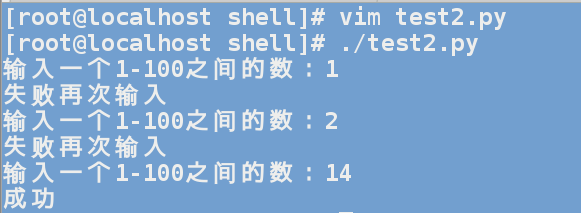9. (if..elif..elif..else考察， 循环语句的考察)写一个带文本菜单的程序,菜单项如下
(1) 取五个数的和
(2) 取五个数的平均 值
....
(X)退出。(exit())

#!/usr/bin/env python
#coding:utf-8

print '''***************功能列表*************** (1)求五个数的和 (2)求五个数的平均值 (3)取五个数中的最大值 (4)取五个数的最小值 (5)退出'''while True:
num = input(" 输入想要使用功能前的序号:")

if num==1:
a = input("输入五个数字：\n")
b = input()
c = input()
d = input()
e = input()
f = a+b+c+d+e
print ("五个数的和为%d") %f

elif num==2:
a =input("输入五个数字：\n")
b =input()
c =input()
d =input()
e =input()
f =(a+b+c+d+e)/5.0
print ("五个数的和为%s") %f
elif num==3:
a =input("输入五个数字：\n")
b =input()
c =input()
d =input()
e =input()
if cmp(a,b)>0:
b = a
if cmp(b,c)>0:
c = b
if cmp(c,d)>0:
d = c
if cmp(d,e)>0:
e = d
print ("五个数中最大的为%s") %e

elif num==4:
a =input("输入五个数字：\n")
b =input()
c =input()
d =input()
e =input()
if cmp(a,b)<0:
b = a
if cmp(b,c)<0:
c = b
if cmp(c,d)<0:
d = c
if cmp(d,e)<0:
e = d
print ("五个数中最小的为%s") %e

elif num==5:
break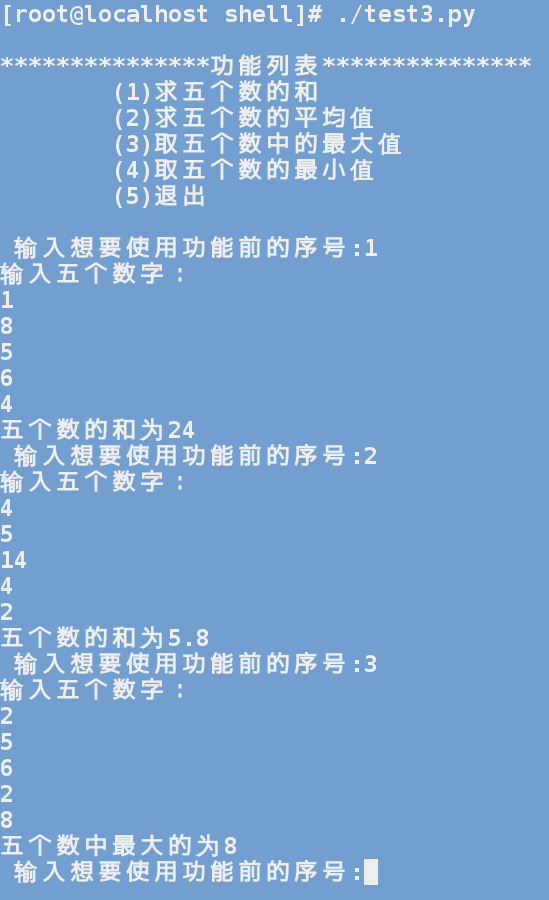10. 有1、2、3、4个数字，能组成多少个互不相同且无重复数字的三位数？都是多少？

#!/usr/bin/env python
#coding:utf-8

'''

'''
i = 0
for x in range(1,5):
for y in range(1,5):
for z in range(1,5):
if (x!=y) and (y!=z) and (z!=x):
i+=1
print("%d%d%d") % (x, y, z),
print("共有%d个满足条件的数字") %i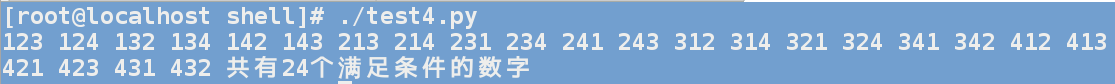2. 在Windows电脑上安装python3版本,并配置环境变量，确保Dos环境下运行脚本;

3. Linux下有多少种运行python的不同方法，并分析各自优缺点。并说说你最喜欢哪一种?2. 在Windows电脑上安装python3版本,并配置环境变量，确保Dos环境下运行脚本;

3. Linux下有多少种运行python的不同方法，并分析各自优缺点。并说说你最喜欢哪一种?4. 编写脚本，使用print语句编写脚本在屏幕上显示你名字、年龄、最喜欢的颜色

©️2019 CSDN 皮肤主题: 大白 设计师: CSDN官方博客